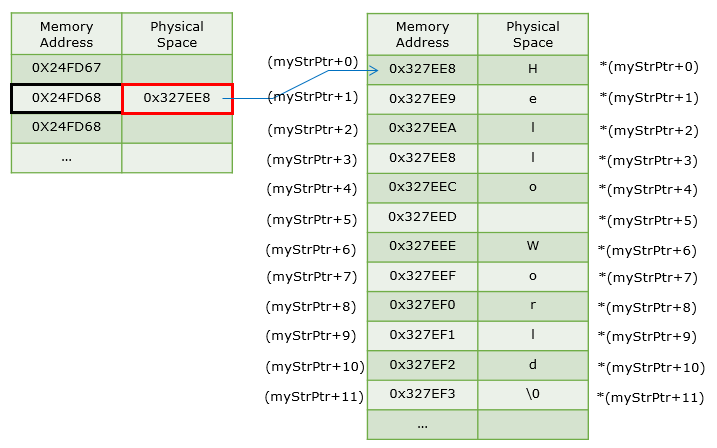C - Pointer                                        Home : www.sharetechnote.com Pointer   Pointer would be one of the most confusing concept not only for those who start learning C programing but also for those who has pretty long experience. It would be one of the tools (concept) that make C language so usefull in many case, but at the same time it might be the one that has caused many problems. Whether you like it or not, I don't think you can avoid the pointer completely if you decided to seriously use C language.       What is Pointer ?   What is the Pointer ?  As you may already know, Pointer is something that points to something else. Just think of such a small laser pointer that you often use during presentation. That pointer is a tool to point to (indicate/refer to) to a specific point on your presentation screen.  Pointer in C is similar to the laser pointer. Pointer in C is also something that points to something else ? Points to what ? The Pointer in C is a tool (operator) that points to (refer to) a specific memory location.   You may roughly guess what the Pointer in C is from this explanation or you may get some better explanation by googling, but you may never understand the concept to the skin unless you use it in your programming. So from now on, I will try to explain on Pointer in examples in various situations.       It would be a little bit easier for you to understand the meaning of Pointers if you understand when people use Pointer in their programming. There is no solid rule on when to use Pointer, but followings are the case where I use Pointers. It may not be clear to you if you don't have any experience with the pointer. So don't worry for now if you don't understand these cases. You would gradually understand as you go through the examples and try things on your own.   i) Create a variable to store an array, but I don't know the exact size of the array for now and the size may change every time when I use it. ii) When I want to pass a certain parameter to a function that can be changed by the operation of the function and want to get the changed value later. (This kind of parameter passing is called 'Pass by Reference')         This example can be the simplest example to show the meaning and usage of a pointer. If you can understand the meaning of each and every line of the code, you can say you have at least basic understandings of Pointer.   #include   int main(int argc, char * argv[]) {     char myVar;     char *myPtr;       myVar = 100;     myPtr = &myVar;       printf("myVar : %#X, %d\n",myVar,myVar);     printf("&myVar : %#X, %d\n",&myVar,&myVar);     printf("myPtr : %#X, %d\n",myPtr,myPtr);     printf("*myPtr : %#X, %d\n",*myPtr,*myPtr);       return 0; }   Can you write down the result of this program without running ? First try writing down the result before you run the program and then run it and compare what you wrote down and the result of the program.   Following is the result of the excution of this program. You may see different values for the parts in blue since the operating system may assign different memory location depending on situations.   Result > myVar : 0X64, 100 &myVar : 0X2FF8C7, 3143879 myPtr : 0X2FF8C7, 3143879 *myPtr : 0X64, 100   For those who are not familiar with how memory allocation works when you run a program I will draw pictures for each of the steps in the source code in terms of memory allocation process.   When you think of memory structure in your PC, just think of you have a spreadsheet (like Microsoft Excel Spreadsheet) and think of each cells in the spreadsheet as a specific memory location in your PC. You may know how to locate (point) to a specific cells in the spreadsheet. You see the column name A,B,C,D ... etc at the top of the spreadsheet and you see raw name 1,2,3,4 ... etc at the left of each raws. Using these column name and raw name, you can specify the specific cells. For example, when I say 'A5', you may know exactaly which cell on the spreadsheet I am referring to. This 'A5' is considered as a kind of address for the specific cell. It means each and every cells on the spreadsheet has its own address. Likewise, each and every memory location in your PC memory has its own address (the exact addressing mechanism is different from Excel spreadsheet, but overall logic is same). Just for convinience, I would use two columns in my diagram.. the second colum represents a specific memory location and the first column represents the address that indicate (point) to the cell.   When you declair a variable as shown below, the operating system reserve a specific memory location and link a label 'myVar' to the location as shown below. (The number/address on the first column in this picture is just arbitrary numbers for explanation. It is highly likely that you would see different numbers when you run the program). At this time, the operating system just reserve a location but it does not put any specific number/data in it since you haven't assigned any specific value to the variable. In most case, the value '0' will be in the location but there may be other garbage number in it.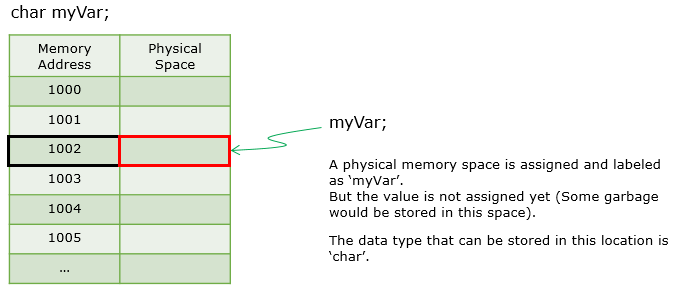Now you assign a value to the variable as shown below. What happens at this point is as shown below. Operating System just put the value to the memory location that the variable (myVar in this case) refers to (points to).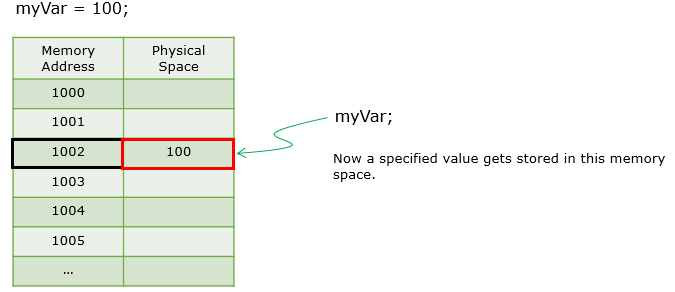Now you declair a variable as a Pointer as shown below. '*' indicate 'this is a Pointer type variable'.  Memory allocation method for the pointer type variable is same as a regular (non pointer type) variable. But the contents (value) stored in the memory is different. In case of pointer variable, the value that will be stored in the location is a 'Memory address'.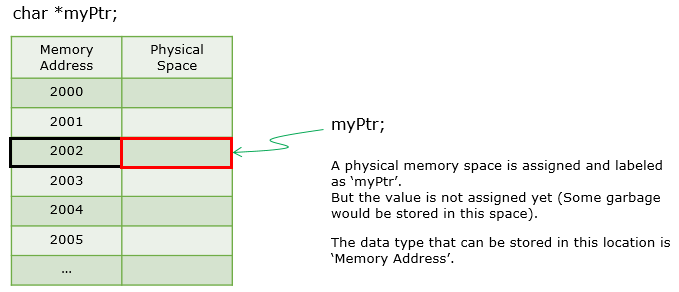Now let's think of how you assign a value to the pointer variable. In some operating system or when you program a very simple chipset, it may be allowed to directly assign a specific value (specific memory address) to the variable. But in most of modern operating system (e.g, Windows), it is not allowed to directly assign the number in user level programming. When you assign a value to a Pointer variable, you have to assign a specific memory address of a specific memory location. The simplest way is to figure out the memory address of an existing variable and assign it to the pointer variable as shown below. The symbol (operator) to figure out a the memory address of a specific variable (non Pointer variable) is '&'.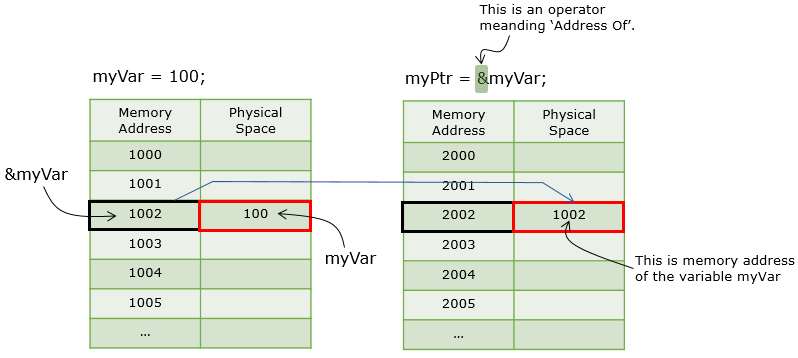Now the last step to use Pointer variable is to figure out the value that is stored at the memory location pointed by the power variable. I know this statement itself sound confusing :)   The answer is very simple, just to use '*' symbol like *myPtr printf("*myPtr : %#X, %d\n",*myPtr,*myPtr). '*myPtr' means 'Get the value stored in the memory location pointed by the address that is stored in the variable myPtr'. I know this sound confusing as well.  How about following picture. I hope this picture would make sense to you.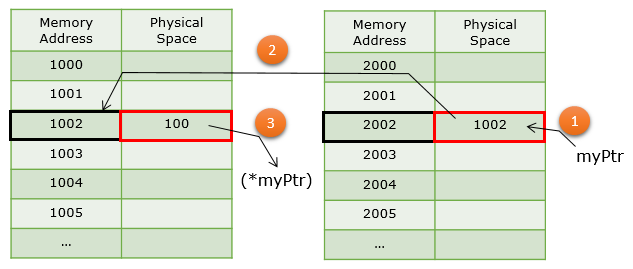I think this model would show you more typical example of Pointer and this would be one of the most typical usage of the Pointer. I recommend you try this example or google similar examples as many as possible until you become confortable with at least this type of pointer operation. First read through the source code and see if you can figure out the result without executing the program first and then go through the result and my explanation.   #include #include #include     int main(int argc, char* argv[]) {     char *myStrPtr;     int i = 0;       myPtr = &myVar;       printf("Address of myStrPtr : %#X\n",&myStrPtr);       myStrPtr=(char *)malloc(20);     printf("myStrPtr (The address value stored at myStrPtr) : %#X\n",myStrPtr);       strcpy(myStrPtr,"Hello World");     printf("A string pointed by myStrPtr : %s\n",myStrPtr);          for(i = 0; i < 11; i++)     {         printf("Address Stored at (myStrPtr+%d),Value pointed by (myStrPtr+%d = %#X) : %c\n",                  i,i,(myStrPtr+i),*(myStrPtr+i));     };       return 0; }     Result > : Following is the result of the program. You may see different values labeled in blue because this is memory address and your system may allocate different locations when you execute the program.   Address of myStrPtr : 0X24FD68 myStrPtr (The address value stored at myStrPtr) : 0X327EE8 A string pointed by myStrPtr : Hello World Address Stored at (myStrPtr+0),Value pointed by (myStrPtr+0 = 0X327EE8) : H Address Stored at (myStrPtr+1),Value pointed by (myStrPtr+1 = 0X327EE9) : e Address Stored at (myStrPtr+2),Value pointed by (myStrPtr+2 = 0X327EEA) : l Address Stored at (myStrPtr+3),Value pointed by (myStrPtr+3 = 0X327EEB) : l Address Stored at (myStrPtr+4),Value pointed by (myStrPtr+4 = 0X327EEC) : o Address Stored at (myStrPtr+5),Value pointed by (myStrPtr+5 = 0X327EED) : Address Stored at (myStrPtr+6),Value pointed by (myStrPtr+6 = 0X327EEE) : W Address Stored at (myStrPtr+7),Value pointed by (myStrPtr+7 = 0X327EEF) : o Address Stored at (myStrPtr+8),Value pointed by (myStrPtr+8 = 0X327EF0) : r Address Stored at (myStrPtr+9),Value pointed by (myStrPtr+9 = 0X327EF1) : l Address Stored at (myStrPtr+10),Value pointed by (myStrPtr+10 = 0X327EF2) : d   Now let's see exactly what's happening when we execute the each line of the code.   The first line to look at is 'char *myStrPtr. With this, a memory location is reserved and allocated to the variable myStrPtr as illustrated below. The memory address shown here based on the execution result on my PC, you may see different numbers when you run on your PC, but the concept is same.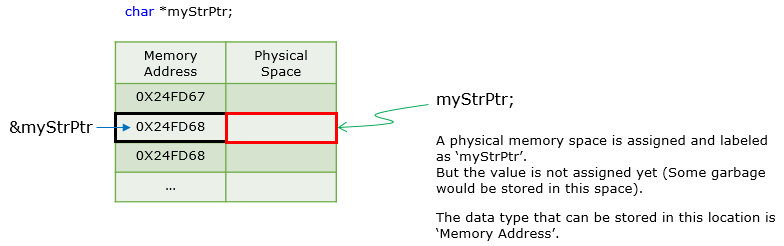Now you do malloc(20) and assign the result to myStrPtr. (Note : Since the return type of malloc is void type, you have to typecast it to char (which is the type of myStrPtr as shown below. As the result, the memory address of the first byte of the area reserved by malloc is assigned to myStrPtr as shown below.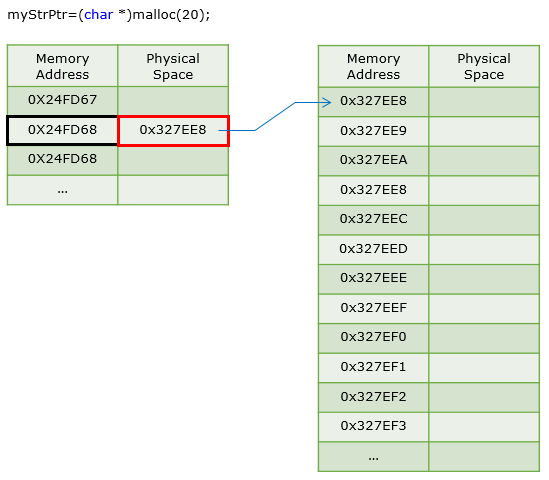Now you put a string to the pointer variable 'myStrPtr'. Since myStrPtr now points to the memory block that is reserved by malloc in previous step, the string "Hello World" are populated into the memory block assined by malloc() as shown below.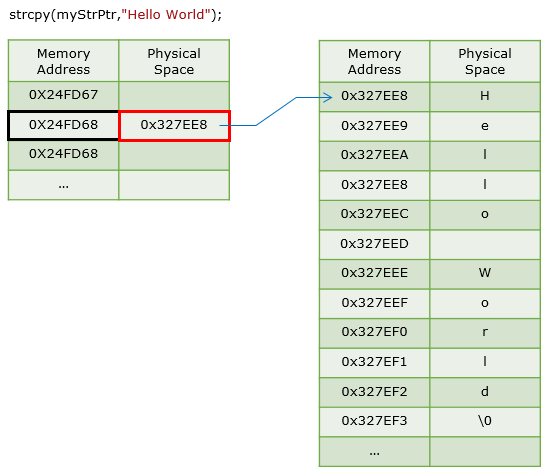Now you do the following loop. I assume that you can understand what this loop do.. the only thing you need to try hard to understand in this loop is to figure out the meaning of following values.. i) (myStrPtr + 0), (myStrPtr + 1), (myStrPtr + 2) ii) *(myStrPtr + 0), *(myStrPtr + 1), *(myStrPtr + 2) I think you would understand this if you carefully read through the first example, but it is understandable if you are still confused :).  With a variable declared as pointer variable, the variable name itself (i.e, the variable name without '*' at the beginning) means 'memory address that is stored in the variable' which points to a specific destination. The variable name with '*' at the beginning (*myStrPtr) means the value stored in the memory address myStrPtr. I know it would still be confusing... before I confuse you even further, I would recommend you to take look at the following illustration. I hope this illustration help you better.       for(i = 0; i < 11; i++)     {         printf("Address Stored at (myStrPtr+%d),Value pointed by (myStrPtr+%d = %#X) : %c\n",                  i,i,(myStrPtr+i),*(myStrPtr+i));     };